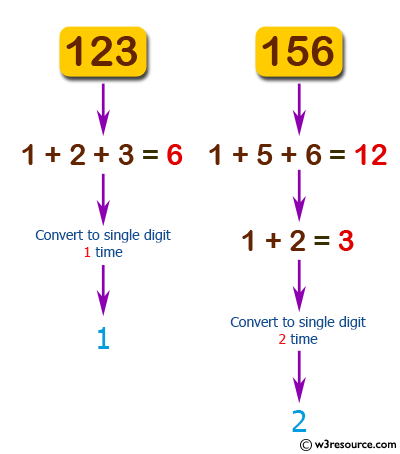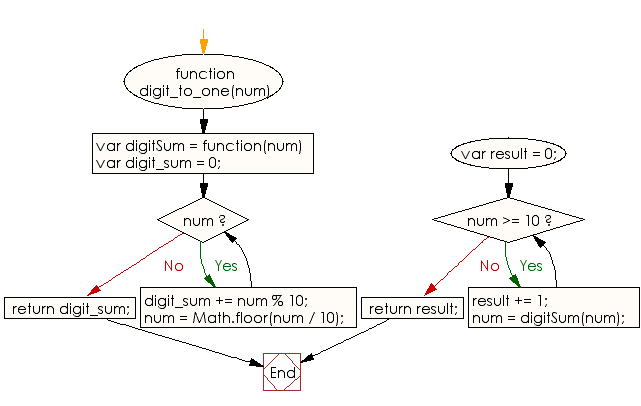# JavaScript: Find the number of times to replace a given number with the sum of its digits until it convert to a single digit number

## JavaScript Basic: Exercise-105 with Solution

Write a JavaScript program to find the number of times to replace a given number with the sum of its digits until it convert to a single digit number.

Pictorial Presentation:Sample Solution:

HTML Code:

``````<!DOCTYPE html>
<html>
<meta charset="utf-8">
<meta name="viewport" content="width=device-width">
<title> Find the number of times to replace a given number with the sum of its digits until it convert to a single digit number</title>
<body>

</body>
</html>
```
```

JavaScript Code:

``````function digit_to_one(num) {

var digitSum = function(num) {

var digit_sum = 0;
while (num) {
digit_sum += num % 10;
num = Math.floor(num / 10);
}

return digit_sum;
};

var result = 0;

while (num >= 10) {
result += 1;
num = digitSum(num);
}

return result;
}

console.log(digit_to_one(123))
console.log(digit_to_one(156))
``````

Sample Output:

```1
2
```

Flowchart:ES6 Version:

``````function digit_to_one(num) {

const digitSum = num => {

let digit_sum = 0;
while (num) {
digit_sum += num % 10;
num = Math.floor(num / 10);
}

return digit_sum;
};

let result = 0;

while (num >= 10) {
result += 1;
num = digitSum(num);
}

return result;
}

console.log(digit_to_one(123))
console.log(digit_to_one(156))
``````

Live Demo:

See the Pen javascript-basic-exercise-105 by w3resource (@w3resource) on CodePen.

What is the difficulty level of this exercise?

Test your Programming skills with w3resource's quiz.

﻿

## JavaScript: Tips of the Day

Returns the sum of the powers of all the numbers from start to end (both inclusive)

Example:

```const sumPower = (end, power = 2, start = 1) =>
Array(end + 1 - start)
.fill(0)
.map((x, i) => (i + start) ** power)
.reduce((a, b) => a + b, 0);
console.log(sumPower(10)); // 385
console.log(sumPower(10, 3)); // 3025
console.log(sumPower(10, 3, 5)); // 2925
```

Output:

```385
3025
2925
```